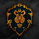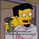3197 lượt xem
This script implements a rebrushed distance-based pair trading strategy. In this strategy, normally they trade the difference between the prices of two instruments. This difference is also called spread. Here, however we’ll trade the difference between two time frames of one instrument. And that's the main trick. Common procedure consists of the following steps:

1. Select two CORRELATED stocks. Here we'll use the same instrument in different TFs.
2. Generate the spread by calculating the difference between the prices/instruments. For distance based pair trading, we need to (rescale the data first and then) check the distance between them.
3. Define the logic to trade the spread and generate the trading signals. In this example we’ll calculate the rolling mean and rolling standard deviation of the spread. Whenever the spread goes above a rolling mean by one standard deviation, we’ll short the spread expecting the mean reversion behavior to hold true. And whenever the spread goes below its rolling mean by one standard deviation, we’ll go long on the spread.

Mind that the meaning of the orange and blue signals depends on whether tf variable is smaller or larger than the built-in timeframe.multiplier variable, i.e. tf of the chart.

Donate: PayPal (paypal.me)

Phát hành các Ghi chú: Added 4 more distance measures.
Phát hành các Ghi chú: Added Mahalanobis distance measure.
Phát hành các Ghi chú: Minor fix.
Phát hành các Ghi chú: Minor fix - typos and norm function.
Phát hành các Ghi chú: Minor fix.

## Bình luậnshort = signal == -1 and signal != -1
plot(short ? diff:na, style=plot.style_circles, color=color.orange, linewidth=3, transp=0)
на графике индиктора ни где нет -1 (( ошибка
Phản hồithis one is fum…!

small typo on line 68, Manhatten needs to be Manhattan
on line 80, need to remove the white space on Correl. change "Correl " to "Correl"
Phản hồi